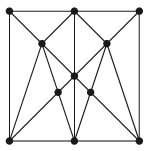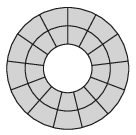Mathematical and Physical Journal
for High Schools
Issued by the MATFUND Foundation
 Already signed up? New to KöMaL?

# KöMaL Problems in Mathematics, December 2019

Show/hide problems of signs:## Problems with sign 'K'

Deadline expired on January 10, 2020.

K. 639. There are 53 passengers in a bus: men, women, girls and boys. The number of women is three times the number of boys, and 10 more than the number of girls. The total number of men and boys is 15. How many men, women, girls and boys are travelling in the bus?

(6 pont)

solution (in Hungarian), statistics

K. 640. The square of a two-digit number ending in 5 can also be calculated as follows: the digit in the tens' place is multiplied by the number greater by 1, and 25 is written after the product. Explain why this method works.

(6 pont)

solution (in Hungarian), statistics

K. 641. Some points are selected in the interior of a convex quadrilateral. These points are connected to each other and to the vertices of the quadrilateral with line segments such that the line segments have no intersection inside the quadrilateral, and they divide the quadrilateral into small triangles and pentagons. (Every interior point is a vertex of some triangle or quadrilateral.) Is it possible to divide the quadrilateral into exactly 2019 polygons in this way?

(6 pont)

solution (in Hungarian), statistics

K. 642. Find all positive integers $\displaystyle x$ and $\displaystyle y$ such that $\displaystyle x^2-y^2=2019$.

(6 pont)

solution (in Hungarian), statistics

K. 643. In the fraction $\displaystyle \frac{a6bc}{de3fg}$ each digit, except 0, occurs exactly once. What may each letter denote if the value of the fraction is $\displaystyle \frac12$?

(6 pont)

solution (in Hungarian), statistics## Problems with sign 'C'

Deadline expired on January 10, 2020.

C. 1574. The points marked in the figure are labelled with the integers $\displaystyle 0$ to $\displaystyle 10$. Then the sum of the numbers on the vertices is written in each triangular region. What are the largest and the smallest possible values of the sum of the 14 numbers obtained in this way?(5 pont)

solution (in Hungarian), statistics

C. 1575. Find all pairs of positive primes $\displaystyle p$, $\displaystyle q$ for which $\displaystyle 2pq+2p-q=q^2-8$.

Proposed by T. Imre, Marosvásárhely

(5 pont)

solution (in Hungarian), statistics

C. 1576. A unit circle is centred at $\displaystyle O$, and $\displaystyle P$ is a point such that $\displaystyle OP=2$. Consider a secant through $\displaystyle P$ that intersects the circle at points $\displaystyle M$ and $\displaystyle N$ such that $\displaystyle M$ bisects the line segment $\displaystyle NP$. Prove that the area of triangle $\displaystyle OMN$ is smaller than $\displaystyle \frac{1}{2}$.

(5 pont)

solution (in Hungarian), statistics

C. 1577. The two and three digit numbers

$\displaystyle \overline{ab},\;\overline{abc},\;\overline{cab}$

(in decimal notation), in this order are three consecutive terms of an increasing, infinite arithmetic sequence. How many terms does this sequence have between 1552 and 2020?

Proposed by B. Bíró, Eger

(5 pont)

solution (in Hungarian), statistics

C. 1578. The circumferences of two congruent rectangles intersect at eight points. Show that the area of the overlapping part of the rectangles is greater than half the area of a rectangle.

(5 pont)

solution (in Hungarian), statistics

C. 1579. Find the real solutions of the equation

$\displaystyle {(x-11)}^{\log_2 (x-10)}= {(x-11)}^{\log_{\frac12}(x-11)}.$

Proposed by B. Bíró, Eger

(5 pont)

solution (in Hungarian), statistics

C. 1580. Barbara places 10 coins on a table in a row at random. In each step, she turns over two adjacent coins. What is the probability that she cannot achieve after a sufficient number of steps, that all coins should have heads'' on top?

(5 pont)

solution (in Hungarian), statistics## Problems with sign 'B'

Deadline expired on January 10, 2020.

B. 5062. Solve the following simultaneous equations:

\begin{align*} x[x]+y[y] & =1,\\ [x]+[y] & =1. \end{align*}

(MI&Q)

(3 pont)

solution (in Hungarian), statistics

B. 5063. In a triangle $\displaystyle ABC$, $\displaystyle BC<AC$ and $\displaystyle \angle ACB$ is a right angle. The tangents drawn from point $\displaystyle A$ to the circle of diameter $\displaystyle BC$ touch it at $\displaystyle C$ and $\displaystyle D$. The line of tangent $\displaystyle AD$ intersects line $\displaystyle BC$ at point $\displaystyle E$. The midpoint of line segment $\displaystyle BC$ is $\displaystyle O$. Prove that the area of triangle $\displaystyle DEO$ equals the area of triangle $\displaystyle AEB$.

Proposed by B. Bíró, Eger

(3 pont)

solution (in Hungarian), statistics

B. 5064. The board'' in the figure consists of 26 fields. In how many different ways is it possible to cover the board with 13 dominoes''? Each domino covers two adjacent fields. (Solutions obtained from each other by rotation are considered different.)(4 pont)

solution (in Hungarian), statistics

B. 5065. The centre of the circumscribed circle of an acute angled triangle $\displaystyle ABC$ is $\displaystyle O$, and the reflections of $\displaystyle O$ in sides $\displaystyle BC$, $\displaystyle CA$ and $\displaystyle AB$ are $\displaystyle O_A$, $\displaystyle O_B$, and $\displaystyle O_C$, respectively. Show that the lines $\displaystyle AO_A$, $\displaystyle BO_B$ and $\displaystyle CO_C$ are concurrent.

(4 pont)

solution (in Hungarian), statistics

B. 5066. Thirty students were taking an exam in a subject called Tautologics''. The students were sitting down in a classroom, and the teacher asked them a single question, How many of the students sitting in this room are going to fail this exam altogether?'' The students had to name a number, one by one, in a row. After each answer, the teacher immediately announced the result which was either pass'' or fail''. When the exam was over, the Student Union requested an inspection by the School District. If it turns out that any student gave the correct answer but still failed the exam, all the results will be cancelled, and everyone will receive a pass'' grade. Is there a strategy for the students to achieve that everyone should pass the exam?

Russian problem

(5 pont)

solution (in Hungarian), statistics

B. 5067. The midpoint of side $\displaystyle AB$ of an acute angled triangle $\displaystyle ABC$ is $\displaystyle F$, and the line $\displaystyle e$ through the point $\displaystyle F$ halves the perimeter of triangle $\displaystyle ABC$. Line $\displaystyle e$ intersects the lines of sides $\displaystyle BC$ and $\displaystyle CA$ at points $\displaystyle D$ and $\displaystyle E$, respectively. Show that the perpendiculars drawn to $\displaystyle AB$ at $\displaystyle F$, to $\displaystyle BC$ at $\displaystyle D$, and to $\displaystyle CA$ at $\displaystyle E$ are concurrent.

(5 pont)

solution (in Hungarian), statistics

B. 5068. Let $\displaystyle p$ be an at most 1998th-degree polynomial such that the values $\displaystyle p(1), p(2),\dots,p(2000)$ form a permutation of the numbers $\displaystyle 1,2,\dots,2000$. Does it follow that $\displaystyle p(1)$ and $\displaystyle p(2000)$ are $\displaystyle 1$ and $\displaystyle 2000$ in some order?

(6 pont)

solution (in Hungarian), statistics

B. 5069. In the quadrilateral $\displaystyle ABCD$, $\displaystyle AB=AD$ and $\displaystyle BC=DC$. Let $\displaystyle M$ be the intersection of the perpendiculars drawn to side $\displaystyle AB$ at $\displaystyle B$, and side $\displaystyle CD$ at $\displaystyle D$. Show that $\displaystyle \angle AMD=\angle BMC$.

(6 pont)

solution (in Hungarian), statistics## Problems with sign 'A'

Deadline expired on January 10, 2020.

A. 764. We call a diagonal of a polygon nice, if it is entirely inside the polygon or entirely outside the polygon. Let $\displaystyle P$ be an $\displaystyle n$-gon with no three of its vertices being on the same line. Prove that $\displaystyle P$ has at least $\displaystyle \frac32(n-3)$ nice diagonals.

Proposed by Bálint Hujter, Budapest and Gábor Szűcs, Szikszó

(7 pont)

statistics

A. 765. Find all functions $\displaystyle f\colon \mathbb{R}\to\mathbb{R}$ which satisfy the following equality for all $\displaystyle x,y\in \mathbb{R}$:

$\displaystyle f(x)f(y)-f(x-1)-f(y+1)=f(xy)+2x-2y-4.$

Proposed by Dániel Dobák, Budapest

(7 pont)

statistics

A. 766. Let $\displaystyle T$ be any triangle such that its side-lengths $\displaystyle a$, $\displaystyle b$, and $\displaystyle c$ and its circumradius $\displaystyle R$ are positive integers. Show that

$\displaystyle a)$ the inradius $\displaystyle r$ of $\displaystyle T$ is a positive integer;

$\displaystyle b)$ the perimeter $\displaystyle P$ of $\displaystyle T$ is a multiple of four; and

$\displaystyle c)$ all three of $\displaystyle a$, $\displaystyle b$, and $\displaystyle c$ are even.

Proposed by Nikolai Beluhov, Bulgaria

(7 pont)

statistics